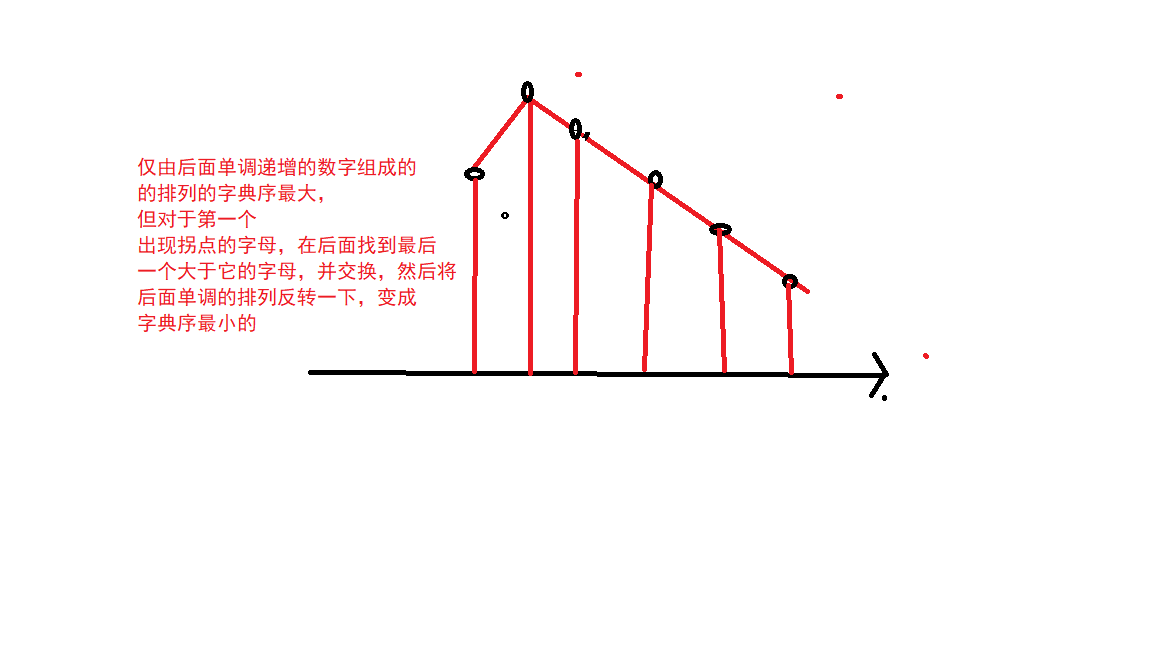## NOIP普及组 火星人(贪心，排列）

2021/1/28 22:32:07 文章标签:

``````数据范围
1≤N≤10000,
1≤M≤100

5
3
1 2 3 4 5

1 2 4 5 3
``````
``````#include<bits/stdc++.h>
using namespace std;
const int N=10010;
int n,m;
int a[N];
int main()
{
cin>>n>>m;
for(int i=1;i<=n;i++) scanf("%d",&a[i]);
while(m--) next_permutation(a+1,a+1+n);
for(int i=1;i<=n;i++) printf("%d ",a[i]);
return 0;
}
``````

2.算法：利用贪心法解决字典序最小相关问题：``````#include<bits/stdc++.h>
using namespace std;
const int N=10010;
int n,m;
int q[N];
int main()
{
cin>>n>>m;
for(int i=1;i<=n;i++) scanf("%d",&q[i]);
while(m--)
{
int k=n-1;
while(q[k]>q[k+1]) k--;
//推出循环时q[k]<q[k+1];
int t=n;
while(q[t]<q[k]&&t>=k) t--;
//int t=k;
//while(t+1<=n&&q[t+1]>q[k]) t++;

swap(q[k],q[t]);

reverse(q+k+1,q+n+1);

}
for(int i=1;i<=n;i++) printf("%d ",q[i]);
return 0;
}
``````

暂无相关的数据...